How to use PropensitySub

In biomedical research, biomarker is an important tool to characterize subpopulations with better/worse disease progression, or different response to a treatment/intervention. When comparing two different treatments, to estimate the treatment benefit within a biomarker subpopulation, ideally the biomarker should be collected in two comparative cohorts with the same procedure - for example, collecting biomarker as a baseline stratification factor in a randomized trial.

However, there are a number of cases where it is impossible to collect biomarker samples from both cohorts. For example, the biomarker positive/negative status is only available in the treatment cohort but not observable in the control cohort. In this case, if the treatment benefit in the biomarker positive group is of interest, one needs to compare the biomarker positive group from the treatment cohort against the “unobserved” biomarker positive group from the control cohort. The “unobserved” biomarker positive group is the subjects who are likely to be biomarker positive if the biomarker is measurable. To predict the “unobserved” biomarker positive group, one may predict the hidden status based on other observed associated features.

This package implements multiple approaches for this type of modeling. In addition to the modeling (session 1), the package also provides handy functions on model fitting diagnostics (session 3 and 4), visualization (session 2, 5), and summarization (session 6).

0. Example Data: dataset “biomarker” from package PropensitySub

• Endpoint: Overall survival (OS, OS.event)
• Treatment Group: “Experimental” and “Control” (Arm)
• Strata: “Positive” and “Negative” (STRATUM)
library(PropensitySub)
library(dplyr)
Patient.ID Sample.ID          Arm Weight ECOG Sex Age        OS OS.event
1     PID040    SID040      Control   40.0    1   F  65  5.059548        0
2     PID112    SID112 Experimental   45.8    2   F  54  2.398357        1
3     PID079    SID079 Experimental   46.0    1   M  41 10.151951        0
STRATUM STRATUM.next
1     <NA>         <NA>
2 Positive     Negative
3 Negative     Negative
#  Control arm subjects have no STRATUM measurments
#  Experimental arm subjects partially miss STRATUM measurement.
table(biomarker$Arm, biomarker$STRATUM, useNA = "ifany")

Negative Positive <NA>
Control             0        0   77
Experimental      115       54    6

1. Estimate treatment effect within each STRATUM.

1.1 Data process: generate a numeric version of strata labeling

Below shows example of 1) imputing missing as negative (assuming missing not at random, and evaluating an extreme case where all missing data are supposed to be negative); 2) treating missing as a separate class: also assuming missing not at random.

indicator_1: numeric version of STRATUM where missing in Experimental Arm is imputed as “Negative”.
indicator_2: numeric version of STRATUM where missing is kept as a seperate stratum

biomarker <- biomarker %>%
mutate(
indicator_1 = case_when(
STRATUM == "Positive" ~ 1,
STRATUM == "Negative" ~ 0,
# impute missing as "Negative" in Experimental Arm
Arm == "Experimental" & is.na(STRATUM) ~ 0,
is.na(STRATUM) & Arm == "Control" ~ -1
),
indicator_2 = case_when(
STRATUM == "Positive" ~ 1,
STRATUM == "Negative" ~ 0,
# keep missing as a seperate stratum in Experimental Arm
Arm == "Experimental" & is.na(STRATUM) ~ 2,
is.na(STRATUM) & Arm == "Control" ~ -1
),
# treatment group needs to be factor
Arm = factor(Arm, levels = c("Control", "Experimental"))

)

1.2 Models for analyzing treatment effect

For subjects in the control arm, to predict a subject’s likelihood of being in different biomarker strata, the first step is calculating P(A | X), where A indicates the biomarker strata and X indicates observed associated features. The model may be learned using the treatment data where both biomarker strata and associated observed features were measured, and be applied in control cohort data to predict the biomarker strata assignments.

Once P(A|X) is calculated, predicted biomarker group of interest in the control cohort can be obtained by inverse probability weighting (IPW) or propensity score matching (PSM). Both IPW and PSM are implemented in the package.

In IPW, the predicted probability of being stratum A will be used as weights when estimating treatment difference of two arms (Hazard ratio for survival endpoint; response rate difference for binary endpoint).

In PSM, the predicted probability of being stratum A will be used for propensity score matching. The matching results will then be used to estimate treatment difference of two arms (Hazard ratio for survival endpoint; response rate difference for binary endpoint).

Below shows different modeling approaches implemented in this package.

1.2.1 plain: binomial/multinomial glm

# plain model with IPW method and aggressive imputation where missing is imputated as negative
ipw_plain_str2 <- ipw_strata(
data.in = biomarker, formula = indicator_1 ~ ECOG + Sex + Age, model = "plain",
indicator.var = "indicator_1", tte = "OS", event = "OS.event", trt = "Arm",
class.of.int = list("Positive" = 1, "Negative" = 0)
)
# get weighted HRs
ipw_plain_str2$stat Coefficient Variance HR CI.Lower CI.Upper Positive -0.4165353 0.3816826 0.6593273 0.2708684 1.6048840 Negative -1.3268812 0.2363171 0.2653034 0.1086968 0.6475431 # check model converge ipw_plain_str2$converged
 TRUE
# get weights
ipw_plain_str2$data %>% dplyr::select(Patient.ID, Arm, STRATUM, ECOG, Sex, Age, indicator_1, pred1, pred0) %>% head() Patient.ID Arm STRATUM ECOG Sex Age indicator_1 pred1 pred0 1 PID040 Control <NA> 1 F 65 -1 0.3312946 0.6687054 2 PID388 Control <NA> 1 M 41 -1 0.2199562 0.7800438 3 PID087 Control <NA> 0 M 37 -1 0.3005256 0.6994744 4 PID134 Control <NA> 0 F 59 -1 0.4266195 0.5733805 5 PID315 Control <NA> 0 M 57 -1 0.3317387 0.6682613 6 PID062 Control <NA> 1 M 42 -1 0.2211980 0.7788020 # plain model with IPW method and missing is kept as another stratum ipw_plain_str3 <- ipw_strata( data.in = biomarker, formula = indicator_2 ~ ECOG + Sex + Age, model = "plain", indicator.var = "indicator_2", tte = "OS", event = "OS.event", trt = "Arm", class.of.int = list("Positive" = 1, "Negative" = 0, "Unknown" = 2) ) # get weighted HRs ipw_plain_str3$stat
Coefficient  Variance        HR   CI.Lower  CI.Upper
Positive  -0.4195829 0.3808727 0.6573210 0.27004073 1.6000210
Negative  -1.4210601 0.2660317 0.2414579 0.09444277 0.6173254
Unknown   -0.4629739 2.6612698 0.6294090 0.09003407 4.4000645
# plain model with PSM method and aggressive imputation
ps_plain_str2 <- ps_match_strata(
data.in = biomarker, formula = indicator_1 ~ ECOG + Sex + Age, model = "plain",
indicator.var = "indicator_1", tte = "OS", event = "OS.event", trt = "Arm",
class.of.int = list("Positive" = 1, "Negative" = 0)
)
# get weighted HRs
ps_plain_str2$stat Coefficient Variance HR CI.Lower CI.Upper Positive -0.5140953 0.2599806 0.5980414 0.2406342 1.4862952 Negative -1.1861074 0.1915910 0.3054078 0.1347383 0.6922601 1.2.2 dwc: doubly weighted control # dwc model with IPW method ipw_dwc <- ipw_strata( data.in = biomarker, formula = indicator_2 ~ ECOG + Sex + Age, model = "dwc", indicator.var = "indicator_2", tte = "OS", event = "OS.event", trt = "Arm", class.of.int = list("Positive" = 1, "Negative" = 0) ) # get weighted HRs ipw_dwc$stat
Coefficient  Variance        HR   CI.Lower  CI.Upper
Positive  -0.4221217 0.3804891 0.6556542 0.26943319 1.5955067
Negative  -1.4201333 0.2660816 0.2416818 0.09452841 0.6179104
# dwc model with PSM method
ps_dwc <- ps_match_strata(
data.in = biomarker, formula = indicator_2 ~ ECOG + Sex + Age, model = "dwc",
indicator.var = "indicator_2", tte = "OS", event = "OS.event", trt = "Arm",
class.of.int = list("Positive" = 1, "Negative" = 0, "Missing" = 2)
)
# get weighted HRs
ps_dwc$stat Coefficient Variance HR CI.Lower CI.Upper Positive -0.3724204 0.2479238 0.6890645 0.28461633 1.6682454 Negative -1.3035235 0.2154364 0.2715732 0.11870059 0.6213281 Missing 0.0000000 2.0000000 1.0000000 0.07255041 13.7835189 1.2.3 wri: weight regression imputation, currently only available with IPW method # data process: create a numeric version of next STRATUM to learn from biomarker <- biomarker %>% mutate( indicator_next_2 = case_when( STRATUM.next == "Positive" ~ 1, STRATUM.next == "Negative" ~ 0, Arm == "Experimental" & is.na(STRATUM.next) ~ 2, is.na(STRATUM.next) & Arm == "Control" ~ -1 ) ) ipw_wri <- ipw_strata( data.in = biomarker, formula = indicator_2 ~ ECOG + Sex + Age, model = "wri", indicator.var = "indicator_2", indicator.next = "indicator_next_2", tte = "OS", event = "OS.event", trt = "Arm", class.of.int = list("Positive" = 1, "Negative" = 0) ) # get weighted HRs ipw_wri$stat
Coefficient  Variance        HR  CI.Lower  CI.Upper
Positive  -0.4361789 0.2539934 0.6465020 0.2764101 1.5121186
Negative  -1.3953601 0.3072783 0.2477438 0.1011774 0.6066276

2. Weighted KM plots from modeling

The treatment benefit within biomarker positive stratum can be calculated as the hazard ratio between the observed biomarker positive group in the treatment cohort against the propensity score matched biomarker positive group in the control cohort. An associated KM can be generated as well. When IPW is used, P(A|X) are used as weights for the HR calculation and KM curves. Binary endpoint is also supported.

# for ipw_plain model results
km_plot_weight(
data.in = ipw_plain_str2$data, indicator.var = "indicator_1", tte = "OS", event = "OS.event", trt = "Arm", class.of.int = list("Positive" = 1, "Negative" = 0) ) []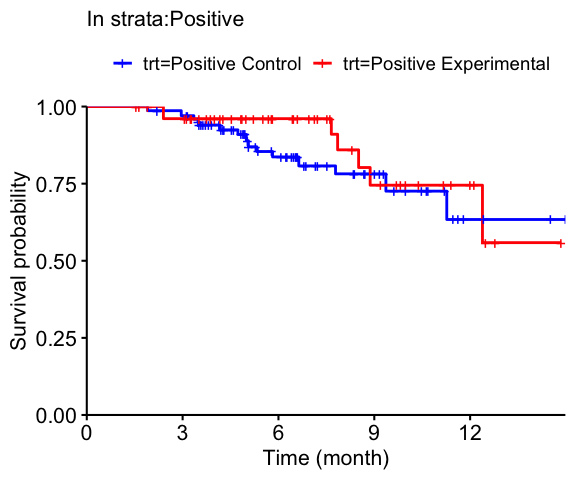[]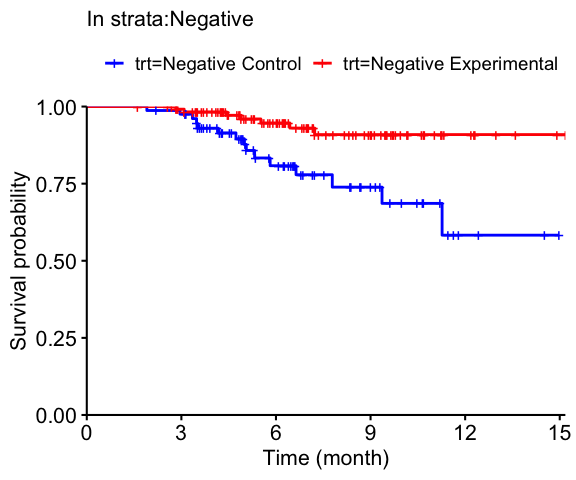# to get weights from model result for further usage ipw_plain_str2$data %>%
dplyr::select(Patient.ID, Arm, STRATUM, ECOG, Sex, Age, indicator_1, pred1, pred0) %>%
Patient.ID     Arm STRATUM ECOG Sex Age indicator_1     pred1     pred0
1     PID040 Control    <NA>    1   F  65          -1 0.3312946 0.6687054
2     PID388 Control    <NA>    1   M  41          -1 0.2199562 0.7800438
3     PID087 Control    <NA>    0   M  37          -1 0.3005256 0.6994744
4     PID134 Control    <NA>    0   F  59          -1 0.4266195 0.5733805
5     PID315 Control    <NA>    0   M  57          -1 0.3317387 0.6682613
6     PID062 Control    <NA>    1   M  42          -1 0.2211980 0.7788020
# for ipw_wri model results
km_plot_weight(
data.in = ipw_wri$data, indicator.var = "indicator_2", tte = "OS", event = "OS.event", trt = "Arm", class.of.int = list("Positive" = 1, "Negative" = 0) ) []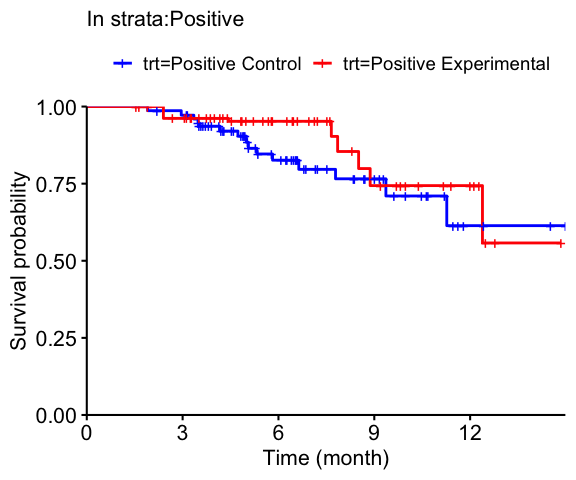[]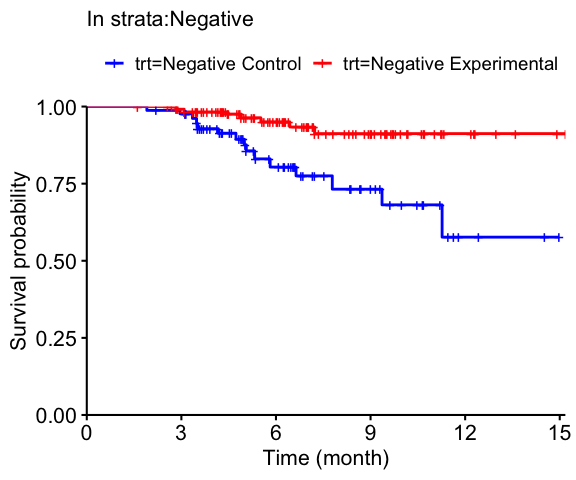# for ps_dwc model results km_plot_weight( data.in = ps_dwc$data,
indicator.var = "indicator_2", tte = "OS", event = "OS.event", trt = "Arm",
class.of.int = list("Positive" = 1, "Negative" = 0, "Missing" = 2)
)
[]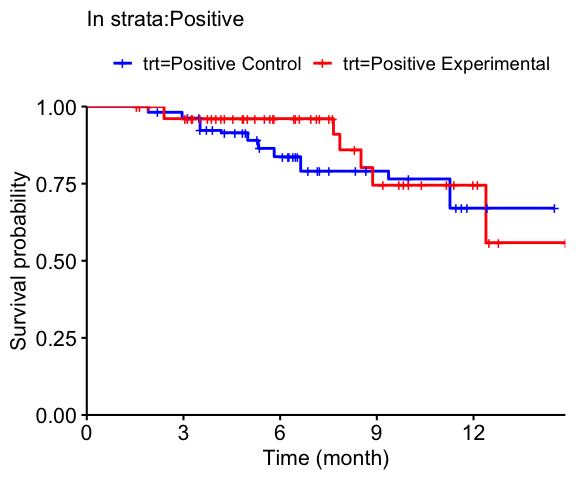[]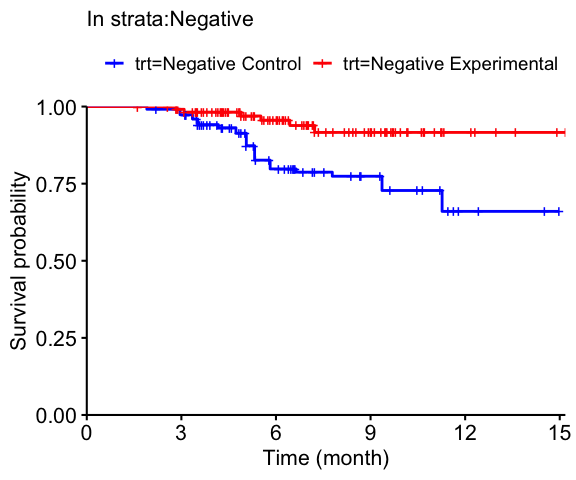[]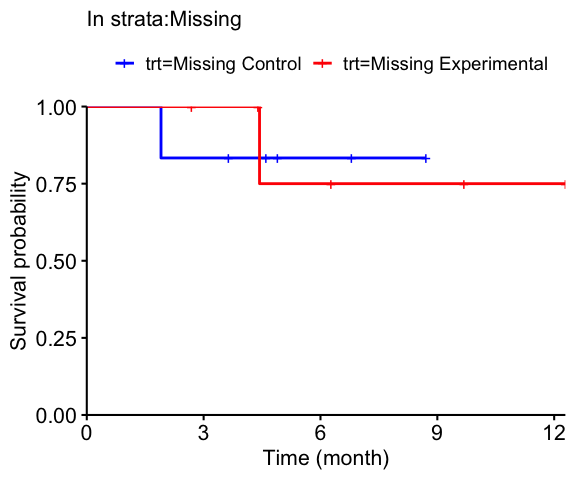3. Check standardized difference of covariates

The package also implemented the absolute standardized mean difference (ASMD) calculation to assess balance of the observed associated features (Xs)(Austin and Stuart, 2015). With a good model fitting, after IPW or PSM adjustment, one would expect a small number of features’ or no feature’s ASMD exceed certain thresholds (such as 0.1 and 0.25). One would expect to see smaller values comparing after-adjustment ASMDs to pre-adjustment ASMDs.

Theoretical calculation is also implemented. The theoretical calculation provides the expected number of features with ASMD greater than a threshold. The calculation depends on sample size and the number of features in X. One may compare the empirical number of features whose ASMD beyond a threshold to the theoretical calculation to assess model performance.

3.1 Example for ipw method with plain model

ipw_plain_diff <- std_diff(
data.in = ipw_plain_str2$data, vars = c("ECOG", "Sex", "Age"), indicator.var = "indicator_1", trt = "Arm", class.of.int = list("Positive" = 1, "Negative" = 0), usubjid.var = "Patient.ID" ) ipw_plain_diff$Positive
var absolute_std_diff                  type
ipw_plain_diff$Negative var absolute_std_diff type 1 ECOG. 0.18839170 adjusted difference 2 Sex.M 0.02334638 adjusted difference 3 Age. 0.12044671 adjusted difference 4 ECOG. 0.25462615 unadjusted difference 5 Sex.M 0.02819748 unadjusted difference 6 Age. 0.12228381 unadjusted difference # Visualize differences std_diff_plot(ipw_plain_diff, legend.pos = "bottom")$Positive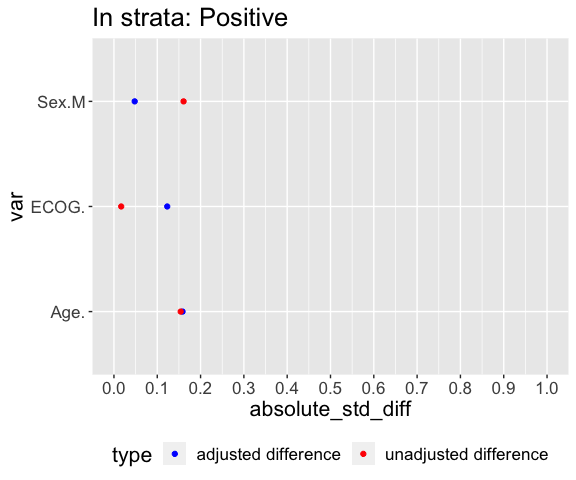$Negative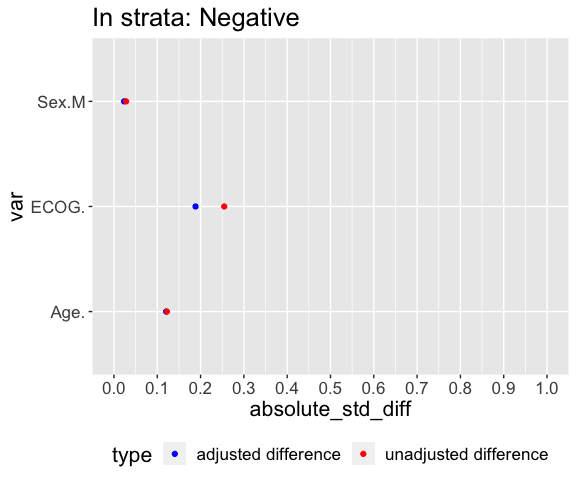3.2 Example for psm method with dwc model ps_dwc_diff <- std_diff( data.in = ps_dwc$data, vars = c("ECOG", "Sex", "Age"),
indicator.var = "indicator_2", trt = "Arm",
class.of.int = list("Positive" = 1, "Negative" = 0, "Missing" = 2),
usubjid.var = "Patient.ID"
)
ps_dwc_diff$Missing var absolute_std_diff type 1 ECOG. 0.0000000 adjusted difference 2 Sex.M 0.0000000 adjusted difference 3 Age. 0.2412968 adjusted difference 4 ECOG. 0.0000000 unadjusted difference 5 Sex.M 0.0000000 unadjusted difference 6 Age. 0.2412968 unadjusted difference # Visualize differences std_diff_plot(ps_dwc_diff)$Positive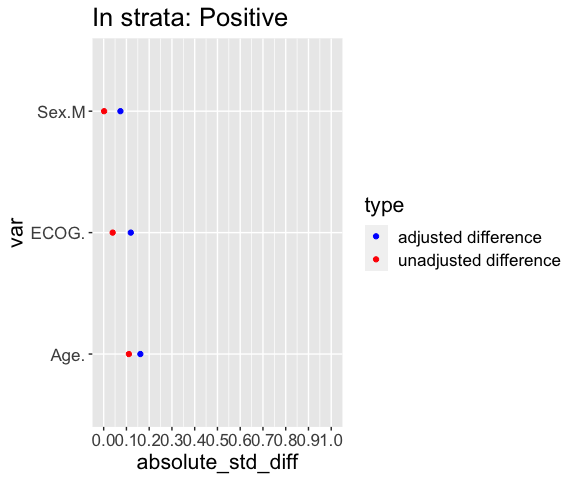$Negative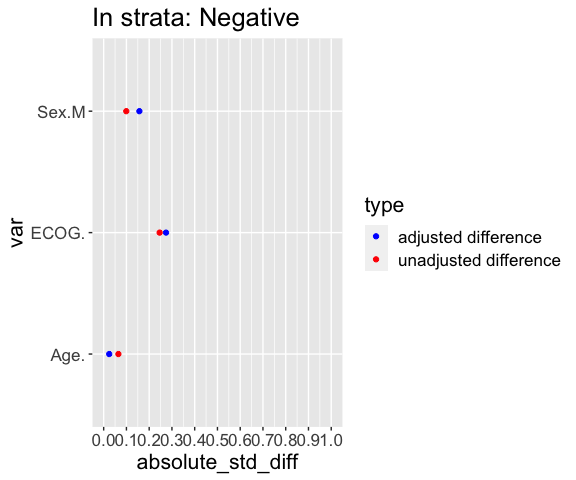$Missing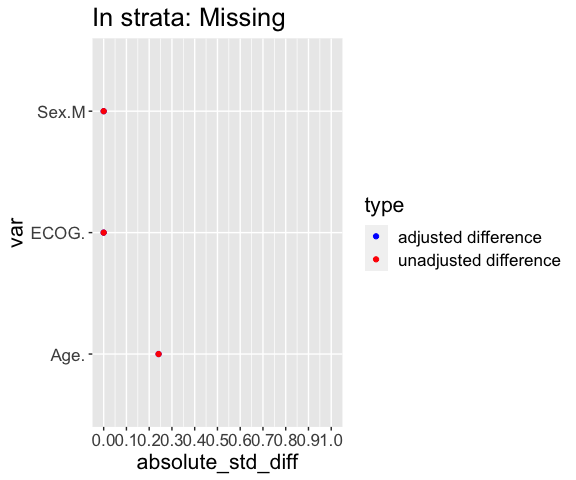4. Expected vs. Observed tables for imbalanced covariates to check model fit

vars <- c("ECOG", "Sex", "Age")
thresholds <- c(0.15, 0.2)
class_int_list <- list("Positive" = 1, "Negative" = 0)
rand_ratio <- 2 # randomization ratio
n_arms <- lapply(class_int_list, function(x) nrow(biomarker %>% filter(indicator_1 %in% x)))
exp_diff <- sapply(n_arms, function(x) {
expected_feature_diff(
n.feature = length(vars),
n.arm1 = x,
n.arm2 = x / rand_ratio,
threshold = thresholds
)
}) %>% t()

# Expected imbalanced features
exp_diff
Expected # features > 0.15 Expected # features > 0.2
Positive                   1.579074                 1.1961231
Negative                   1.026179                 0.6170036
# Calculate the observed imbalanced features in model ipw_plain_diff
obs_diff_cnt <- sapply(thresholds, function(th){
sapply(ipw_plain_diff, function(gp){
ft <- as.character(subset(gp, type=="adjusted difference" & absolute_std_diff>=th)$var) length(unique(sapply(ft, function(ff)strsplit(ff, split="\\.")[]))) }) }) colnames(obs_diff_cnt) <- paste0("Observed # features > ", thresholds) rownames(obs_diff_cnt) <- names(ipw_plain_diff) # the number of observed imbalanced features for each threshold # Compare expected to observed # of imbalanced features to check model fit obs_diff_cnt Observed # features > 0.15 Observed # features > 0.2 Positive 1 0 Negative 1 0 obs_diff_fac <- sapply(thresholds, function(th) { sapply(ipw_plain_diff, function(gp) { ft <- as.character(subset(gp, type=="adjusted difference" & absolute_std_diff>=th)$var)
paste(ft, collapse=",")
})
})
colnames(obs_diff_fac) <- paste0("features > ", thresholds)
rownames(obs_diff_fac) <- names(ipw_plain_diff)
# the observed individual features that are imbalanced for each threshold
obs_diff_fac
features > 0.15 features > 0.2
Positive "Age."          ""
Negative "ECOG."         ""

5. Bootstrap to get a robust CIs for the treatment effect

5.1 Example for ipw method with plain model

boot_ipw_plain <- bootstrap_propen(
data.in = biomarker, formula = indicator_1 ~ ECOG + Sex + Age,
indicator.var = "indicator_1", tte = "OS", event = "OS.event", trt = "Arm",
class.of.int = list("Positive" = 1, "Negative" = 0),
estimate.res = ipw_plain_str2, method = "ipw", n.boot = 100
)
# get bootstrap CI
boot_ipw_plain$est.ci.mat Estimate Bootstrap CI low Bootstrap CI high Positive -0.4165353 -1.283337 0.4502662 Negative -1.3268812 -2.271757 -0.3820054 HR ratio: strata over Positive Positive 1.000000 Negative 0.402385 # summary statistics from bootstraps boot_ipw_plain$boot.out.est
Mean         Var     Median          Min
Positive;Coefficient   -0.3540341 0.195588381 -0.3698299 -1.683188353
Positive;Variance       0.4310293 0.013133483  0.4027040  0.243995859
Positive;HR             0.7741164 0.134388387  0.6908575  0.185780696
Positive;CI.Lower       0.3123257 0.022428198  0.2830601  0.048395835
Positive;CI.Upper       1.9439027 0.911859777  1.6892788  0.693508873
Negative;Coefficient   -1.3246478 0.232409179 -1.2919873 -3.835899987
Negative;Variance       0.2752179 0.012857275  0.2478241  0.145512877
Negative;HR             0.2926887 0.014533128  0.2747243  0.021581906
Negative;CI.Lower       0.1186285 0.002994016  0.1128095  0.002791294
Negative;CI.Upper       0.7330693 0.077532519  0.6865619  0.166868400
Positive over Positive  1.0000000 0.000000000  1.0000000  1.000000000
Negative over Positive  0.4372193 0.061767413  0.4234440  0.042909461
Max     VarLog
Positive;Coefficient    0.9218116         NA
Positive;Variance       0.8232510 0.06302566
Positive;HR             2.5138404 0.19558838
Positive;CI.Lower       0.9368164 0.23989078
Positive;CI.Upper       6.7456048 0.18402106
Negative;Coefficient   -0.3512042         NA
Negative;Variance       1.0681811 0.08751756
Negative;HR             0.7038400 0.23240918
Negative;CI.Lower       0.2927817 0.38615749
Negative;CI.Upper       1.6920143 0.14463584
Positive over Positive  1.0000000 0.00000000
Negative over Positive  1.9730948 0.32326143
# error status and convergence status
boot_ipw_plain$error.est  0 boot_ipw_plain$conv.est
 TRUE TRUE TRUE TRUE TRUE TRUE TRUE TRUE TRUE TRUE TRUE TRUE TRUE TRUE TRUE
 TRUE TRUE TRUE TRUE TRUE TRUE TRUE TRUE TRUE TRUE TRUE TRUE TRUE TRUE TRUE
 TRUE TRUE TRUE TRUE TRUE TRUE TRUE TRUE TRUE TRUE TRUE TRUE TRUE TRUE TRUE
 TRUE TRUE TRUE TRUE TRUE TRUE TRUE TRUE TRUE TRUE TRUE TRUE TRUE TRUE TRUE
 TRUE TRUE TRUE TRUE TRUE TRUE TRUE TRUE TRUE TRUE TRUE TRUE TRUE TRUE TRUE
 TRUE TRUE TRUE TRUE TRUE TRUE TRUE TRUE TRUE TRUE TRUE TRUE TRUE TRUE TRUE
 TRUE TRUE TRUE TRUE TRUE TRUE TRUE TRUE TRUE TRUE

5.2 Example for ipw method with wri model

boot_ipw_wri <- bootstrap_propen(
data.in = biomarker, formula = indicator_2 ~ ECOG + Sex + Age,
indicator.var = "indicator_2", indicator.next = "indicator_next_2",
tte = "OS", event = "OS.event", trt = "Arm",
class.of.int = list("Positive" = 1, "Negative" = 0),
estimate.res = ipw_wri, method = "ipw", n.boot = 100
)
# get bootstrap CI
boot_ipw_wri$est.ci.mat Estimate Bootstrap CI low Bootstrap CI high Positive -0.4361789 -1.332213 0.4598551 Negative -1.3953601 -2.492321 -0.2983993 HR ratio: strata over Positive Positive 1.0000000 Negative 0.3832065 5.3 Example for psm method with dwc model boot_ps_dwc <- bootstrap_propen( data.in = biomarker, formula = indicator_2 ~ ECOG + Sex + Age, indicator.var = "indicator_2", tte = "OS", event = "OS.event", trt = "Arm", class.of.int = list("Positive" = 1, "Negative" = 0), estimate.res = ps_dwc, method = "ps", n.boot = 100 ) # get bootstrap CI boot_ps_dwc$est.ci.mat
Estimate Bootstrap CI low Bootstrap CI high
Positive -0.3724204        -1.715192       0.970351514
Negative -1.3035235        -2.600825      -0.006221976
HR ratio: strata over Positive
Positive                      1.0000000
Negative                      0.3941187

6. Create forestplot to visualize multiple model results

boot_models <- list(
ipw_plain = boot_ipw_plain,
ipw_wri = boot_ipw_wri,
ps_dwc = boot_ps_dwc
)
cols <- c("Estimate", "Bootstrap CI low", "Bootstrap CI high")
# get HRs and bootstrap CIs
boots_dp <- lapply(boot_models, function(x){
cis <- x\$est.ci.mat[ , cols, drop = FALSE] %>%
exp() %>% round(2) %>% as.data.frame()
colnames(cis) <- c("HR", "LOWER", "UPPER")
cis %>% mutate(
Group = rownames(cis)
)
})
boots_dp <- do.call(rbind, boots_dp) %>%
mutate(
Methods = rep(c("IPW plain", "IPW wri", "PS dwc"), 2),
Methods_Group = paste(Methods, Group),
Group = factor(Group, levels = c("Positive", "Negative")),
Methods = factor(Methods, levels = c("IPW plain", "IPW wri", "PS dwc"))
) %>%
arrange(Methods, Group)
forest_bygroup(
data = boots_dp, summarystat = "HR", upperci = "UPPER", lowerci = "LOWER",
population.name = "Methods_Group", group.name = "Methods",
color.group.name = "Group",
stat.label = "Hazard Ratio",
stat.type.hr = TRUE, log.scale = FALSE,
endpoint.name = "OS", study.name = "Example Study", draw = TRUE
)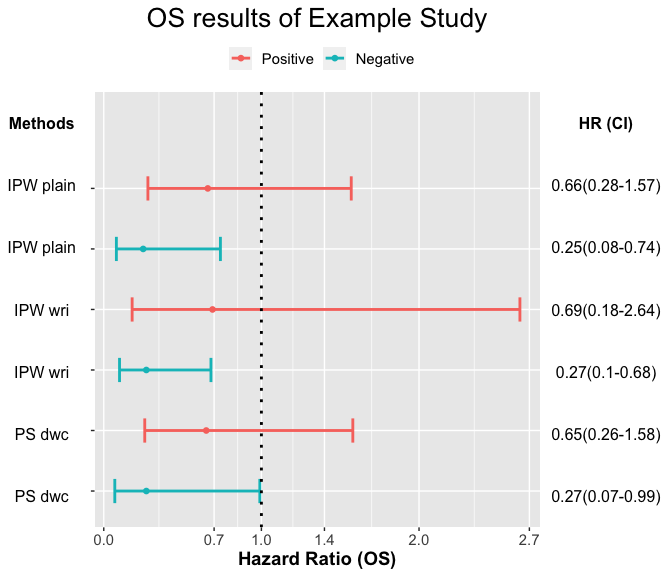7. Functions

Below are the main functions in this package :

• ipw_strata(): IPW, allows for 2 or multiple classes. It also allows for predicting both patients with unknown class label in control arm and patients with unknown class label in trt arm. Supports both survival endpoint (HR as effect size estimator) and response endpoint (ORR difference as effect size estimator)

• ps_match_strata(): PSM, similar to ipw_strata()

• km_plot_weight(): weighted km, can take outputs from either ipw_strata() or ps_match_strata()

• bootstrap_propen(): bootstrap function; can take outputs from both ipw_strata() and ps_match_strata(); outputs bootstrap CI as well as summary statistics (median, mean, etc) of bootstrap HRs (ORR deltas)

• forest_bygroup(): forestplot function; additional functionality to impose extra summary statistics on forestplot (e.g. median bootstrap HR on top of HR point estimate)

• std_diff(): Calculate feature’s standardized difference across two arms. This function can be used to check covariate balance between the (matched) strata from two arms.

• expected_feature_diff(): Calculate expected number of features showing standardized difference > threshold. This function can be used to assess whether the feature balance in the matched/adjusted data set is comparable to an RCT.